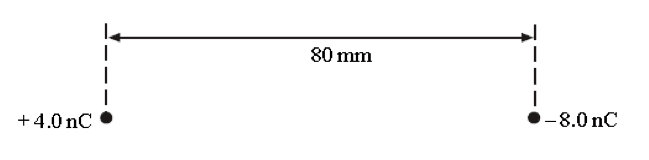# Help with determining distance where electric potential is 0

• Hannah7h

#### Hannah7h

Member advised to use the homework template for posts in the homework sections of PF.
Question states 'Determine the distance from the +4.0nC charge to the point, along the straight line between the charges, where the electric potential is zero.'This is as far as I've got: so I've used V=Q/4pi(epsilon0)r and then set it so V1+V2=0 (to find the point where the resultant electric potential is zero). Then the 4pi(epsilon0) can just be ignored as it is a constant, therefore V1=Q1/R1 and then V2=Q2/R2 therefore Q1/R1 + Q2/R2 = 0 so then (substituting in the values), 4x10^-9/r - 8x10^-9/ (80x10^-3) -r = 0 AND now I'm not sure where to go from this point

Thank you for any help